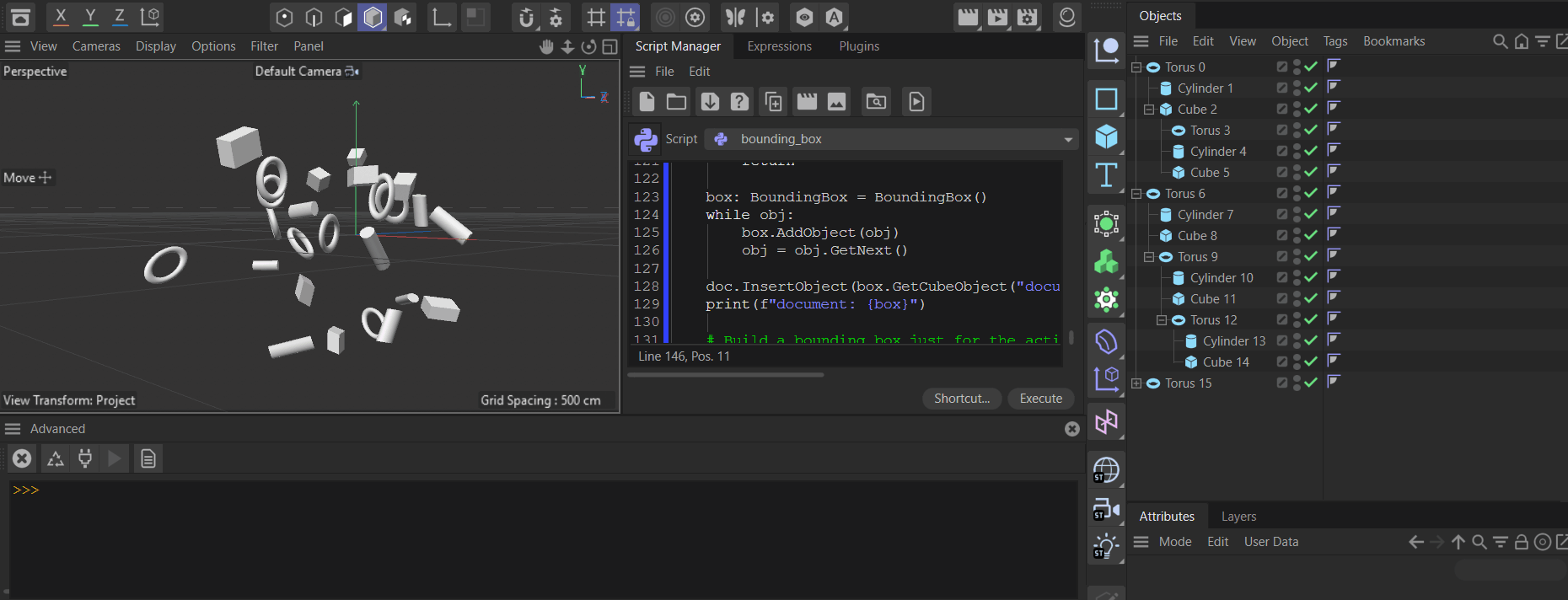# Unsolved How to get the bounding box for the whole scene

I want to get the highest and lowest world position( pos.Y of Highest point and lowest point for the whole scene.
Is there a quick method to get it apart from for looping all objects in the scene?

Hello @sawyerwolf19,

Thank you for reaching out to us and please excuse the slight delay.

Cinema 4D offers bounding box computation via BaseObject.GetRad() and BaseObject.GetMp(). `GetMp` returns here the offset of the midpoint of the bounding from the origin of the object (usually the null vector for primitive generators) and `GetRad` the distance from the midpoint to the boundary of the bounding box on each principal axis.A `BaseObject` will always express its bounding box in its local object space. And a `BaseObject` will also not include its descendants in its bounding box computation. A null object will for example always return `(0, 0, 0)` for its radius and midpoint.

So, one must carry out such computations oneself, but iterating over points is not necessary, one can reuse the mentioned `BaseObject` methods. Your question was slightly ambiguous, as you did not express in which space you want the cumulative bounding boxes to be expressed: in the product of the spaces of all contained objects or in world space.

I have provided a solution for world space below, as I assumed this to be more likely to be what you want.

Cheers,
Ferdinand

Result:Code

``````"""Demonstrates building bounding boxes in world space.

Must be run as a Script Manger script. Will build a bounding box for all objects in a scene and
one for the current object selection.
"""

import c4d
import typing
import sys

op: typing.Optional[c4d.BaseObject]  # The active object, can be `None`.
doc: c4d.documents.BaseDocument  # The active document.

class BoundingBox:
"""Represents a bounding box in the world space of a document.

Other than for BaseObject.GetRad(), this bounding box will not be aligned with the local
transform of an object. Instead, the orientation of BoundingBox will always be equal to the
identity matrix, i.e., world space.
"""
def __init__(self) -> None:
"""Initializes the bounding box.
"""
self.min: c4d.Vector = c4d.Vector(sys.float_info.max)
self.max: c4d.Vector = c4d.Vector(-sys.float_info.max)

def __repr__(self) -> str:
"""Returns a string representation of the bounding box.
"""
m, r = ((round(m.x, 3), round(m.y, 3), round(m.z, 3)),
(round(r.x, 3), round(r.y, 3), round(r.z, 3)))
return (f"{self.__class__.__name__} with the midpoint {m} and radius {r}.")

def AddPoint(self, p: c4d.Vector) -> None:
"""Adds the point #p to the bounding box.

#p must be in global space and the bounding box will be updated so that it includes #p.
"""
if p.x < self.min.x:
self.min.x = p.x
if p.y < self.min.y:
self.min.y = p.y
if p.z < self.min.z:
self.min.z = p.z
if p.x > self.max.x:
self.max.x = p.x
if p.y > self.max.y:
self.max.y = p.y
if p.z > self.max.z:
self.max.z = p.z

def AddObject(self, node: c4d.BaseObject) -> None:
"""Adds the object #node and all its descendants to the bounding box.

The bounding box will be updated so that it encompasses #node and its descendants.
"""
transform: c4d.Matrix = node.GetMg()
midpoint: c4d.Vector = node.GetMp()

for child in node.GetChildren():
box = BoundingBox()
childMidpoint: c4d.Vector  = box.GetMidpoint()

def GetMidpoint(self) -> c4d.Vector:
"""Returns the midpoint of the bounding box in global space.
"""
return (self.min + self.max) * 0.5

"""Returns the distance from the midpoint of the bounding box to its boundary on each axis.
"""
return self.max - self.GetMidpoint()

def GetCubeObject(self, name: typing.Optional[str] = None) -> c4d.BaseObject:
"""Returns a cube generator object representation of this bounding box.
"""
cube: c4d.BaseObject = c4d.BaseObject(c4d.Ocube)
if not cube:
raise MemoryError(f"{cube = }")

tag: c4d.BaseTag = cube.MakeTag(c4d.Tdisplay)
if not tag:
raise MemoryError(f"{tag = }")

cube.SetMg(c4d.Matrix(off=self.GetMidpoint()))
tag[c4d.DISPLAYTAG_AFFECT_DISPLAYMODE] = True
if isinstance(name, str):
cube.SetName(name)

return cube

def main() -> None:
"""Runs the example.
"""
# Build the bounding box for the whole document by adding each top level object to the box.
obj: c4d.BaseObject = doc.GetFirstObject()
if obj is None:
return

box: BoundingBox = BoundingBox()
while obj:
obj = obj.GetNext()

doc.InsertObject(box.GetCubeObject("document_box"))
print(f"document: {box}")

# Build a bounding box just for the active selection.
selection: list[c4d.BaseObject] = doc.GetActiveObjects(c4d.GETACTIVEOBJECTFLAGS_NONE)
if len(selection) < 1:
return

box: BoundingBox = BoundingBox()
for obj in selection:

doc.InsertObject(box.GetCubeObject("selection_box"))
print(f"selection: {box}")

if __name__ == "__main__":
main()
``````

edit: fixed upper boundary bug

MAXON SDK Specialist
developers.maxon.net

Thank you !
This was on my to do list aswell because i want to frame/place cameras reasonably in a scene ... neglegted it for a long time ... now i just need to implement it ...Follow up question is it possible to exclude the world origin from the list of points?
If you place the objects outside the "Center" the world origin is included as a "point" hence the bounding box extends to the world Origin.

I am not sure where in the codec c4d.Vector(0,0,0) is set

Hey @mogh,

Thank you for pointing out that problem. No, there is no `Vector(0, 0, 0)` being added, there was simply a small bug in my code.

``````self.min: c4d.Vector = c4d.Vector(sys.float_info.max)
self.max: c4d.Vector = c4d.Vector(sys.float_info.min)
``````

`float_info.min` is `2.2250738585072014e-308`, i.e., the smallest representable increment of the float type, not the lower representable boundary. So, the code did initialize the max-value boundary as ~(0, 0, 0) which then caused values to be discarded which should not be discared. I of course meant here the following:

``````self.min: c4d.Vector = c4d.Vector(sys.float_info.max)
self.max: c4d.Vector = c4d.Vector(-sys.float_info.max)
``````

Fixing this should fix your problems. I have updated my original posting to not mislead future readers.

Cheers,
Ferdinand

MAXON SDK Specialist
developers.maxon.net